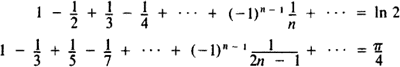# Alternating Series

(redirected from Alternating sum)

## alternating series

[′ȯl·tər·nād·iŋ ′sir·ēz]
(mathematics)
Any series of real numbers in which consecutive terms have opposite signs.
McGraw-Hill Dictionary of Scientific & Technical Terms, 6E, Copyright © 2003 by The McGraw-Hill Companies, Inc.
The following article is from The Great Soviet Encyclopedia (1979). It might be outdated or ideologically biased.

## Alternating Series

an infinite series whose terms are alternately positive and negative:

u1 - u2 + u3 - u4 + … + (-I)n-1un + …

for uk > 0. If the terms of an alternating series monotonically decrease (un+1 < un) and tend toward zero (lim un = 0), then the series is convergent (the Leibniz theorem). The remainder of the convergent series

rn = (-1)nun+1 + …

has the sign of its first term and is less than this term in absolute value. Some very simple examples of convergent alternating series areReferences in periodicals archive ?
Then we use the expression for the power sum polynomial as an alternating sum of hook Schur polynomials.
We next rewrite the power sum symmetric function as an alternating sum of hook Schur functions
We push forward the resulting alternating sum to [[LAMBDA].sub.k,n], and then consider the class in q[H.sup.*](Gr(k, n)).
The main ingredient is Rice's formula Flajolet and Sedgewick (1995) which allows to write an alternating sum as a contour integral:
For the asymptotic evaluation, we use a contour integral representation of alternating sums ("Rice's method").
Proof of Theorem 2: The exactness of the sequence (1) gives us that the alternating sum of dimensions is 0.
The terms [alpha] and [beta] are typical expressions to which 'Rice's method' can be applied, due to the presence of the binomial coefficient inside an alternating sum. First we deal with a, and the function involved for Rice's method is f(z) = 1/[Q.sup.z-1]-1.
Now we look at the alternating sum labelled (b) which has a double pole at z = 1 and a simple pole at z = 2.
Now Rice's method can again be used to approximate the alternating sums. First we deal with part (a) by considering the poles of the function [n;z] f(z) with f(z) = [Q.sup.z]-1/1-[Q.sup.z-2].
Turker, "Alternating sums of the powers of Fibonacci and Lucas numbers," Miskolc Mathematical Notes, vol.
Simsek, "A note on the alternating sums of powers of consecutive q-integers," Advanced Studies in Contemporary Mathematics, vol.
They cover initial encounters with combinatorial reasoning; selections, arrangements, and distributions; binomial series and generating functions; alternating sums, the inclusion-exclusion principle, rook polynomials, and Fibonacci Nim; recurrence relations; special numbers; linear spaces and recurrence sequences; and counting with symmetries.

Site: Follow: Share:
Open / Close